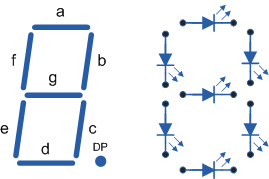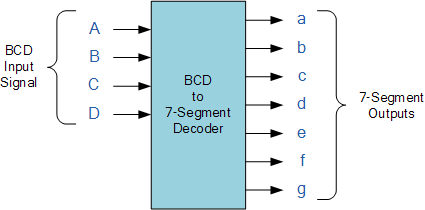# BCD to Seven-Segment Decoder Program in VHDL

This decoder takes as its input four bits that represent a binary coded (BCD) value and generates a 7-bit output to drive a seven-segment LED.

Each segment is a small LED, which glows when driven by an electrical signal. The segments are labelled from a to g as shown in the figure below:VHDL Program for BCD to Seven Segment Decoder:

```library ieee;
use ieee.std_logic_1164.all;

entity seg7 is
port (bcd : in std_logic_vector(3 downto 0);
leds : out std_logic_vector(1 to 7));
end seg7;

architecture behaviour of seg7 is
begin
process (bcd)
begin
case bcd is
when “0000” => leds <= “1111110”;
when “0001” => leds <= “0110000”;
when “0010” => leds <= “1101101”;
when “0011” => leds <= “1111001”;
when “0100” => leds <= “0110011”;
when “0101” => leds <= “1011011”;
when “0110” => leds <= “1011111”;
when “0111” => leds <= “1110000”;
when “1000” => leds <= “1111111”;
when “1001” => leds <= “1110011”;
when others => leds <= “-------”;
end case;
end process;
end behaviour;```

This site uses Akismet to reduce spam. Learn how your comment data is processed.

error: